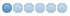# Running on Raspberry Pi2 and learning some BASIC Linux

• doogie
• Offline
• New Member
•1 year 9 months ago #8826 by doogie
I know this is old, but for anyone else who comes looking for how to fix this, it's in db.c in fread_string(). Sometimes, there is a ~ stored in the memory location in front of tmp array. The for loop will decrement below the starting memory address, making it read the ~ and think it's at the end of the room. So it expects to start a new room, but is in the middle of a room record which makes it error out. This seems to be related somehow to how the Pi stores data, although it could technically affect any platform.

Anyway, the fix is to modify the for loop to add a pointer check:
for (point-- ; (*point=='\r' || *point=='\n') && point > tmp; point--);

and add an if check to the else statement after the loop to make sure we're pointing at the right place:
if (*point == '\n' || *point == '\r')
*point = '\r';
else
*(++point) = '\r';

*(++point) = '\n';
*(++point) = '\0';

That should stop the crashing. I believe this is slotted to be fixed soon.

Cheers

• criz
• Topic Author
• Offline
• New Member
•1 year 4 months ago #9771 by criz
Hello after 5 years!

I tried this with a new install: I'm getting a error on running bin/circle -m

Dec 19 14:26:51 2020 :: SYSERR: fread_string: string too large (db.c)
Dec 19 14:26:51 2020 :: trig vnum 0

Here's the segment of the db.c file I updated. Did I miss something?

if (!fgets(tmp, 512, fl)) {
log("SYSERR: fread_string: format error at or near %s", error);
exit(1);
}
/* If there is a '~', end the string; else put an "\r\n" over the '\n'. */
/* now only removes trailing ~'s -- Welcor */
point = strchr(tmp, '\0');
for (point-- ; (*point=='\r' || *point=='\n') && point > tmp; point--);
if (*point == '\n' || *point == '\r') {
*point = '\r';
} else {
*(++point) = '\r';
*(++point) = '\n';
*(++point) = '\0';
}
templength = point - tmp;

if (length + templength >= MAX_STRING_LENGTH) {
log("SYSERR: fread_string: string too large (db.c)");
log("%s", error);
exit(1);
} else {
strcat(buf + length, tmp); /* strcat: OK (size checked above) */
length += templength;
}
} while (!done);

• doogie
• Offline
• New Member
•1 year 4 months ago #9772 by doogie
At a glance, it looks like you may have inadvertantly deleted the tilde check. It should look something like this:

/* If there is a '~', end the string; else put an "\r\n" over the '\n'. */
/* now only removes trailing ~'s -- Welcor */
point = strchr(tmp, '\0');
for (point-- ; (*point=='\r' || *point=='\n') && point > tmp; point--);
if (*point=='~') {
*point='\0';
done = 1;
} else {
if (*point == '\n' || *point == '\r')
*point = '\r';
else
*(++point) = '\r';

*(++point) = '\n';
*(++point) = '\0';
}

templength = point - tmp;

if (length + templength >= MAX_STRING_LENGTH) {
log("SYSERR: fread_string: string too large (db.c)");
log("%s", error);
exit(1);
} else {
strcat(buf + length, tmp); /* strcat: OK (size checked above) */
length += templength;
}
} while (!done);

Best,
Doogie
The following user(s) said Thank You: lacrc

• criz
• Topic Author
• Offline
• New Member
•1 year 3 months ago #9779 by criz
Just following up - thank you to the kind person who updated the relevant file on github! Downloaded the zip file from there, compiled and have it successfully running on a Raspberry Pi 2 - no errors! Happy to be back in Midgaard !

•